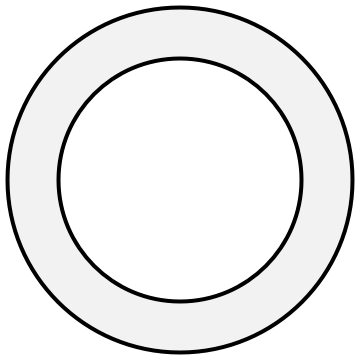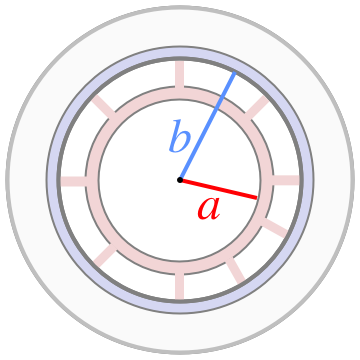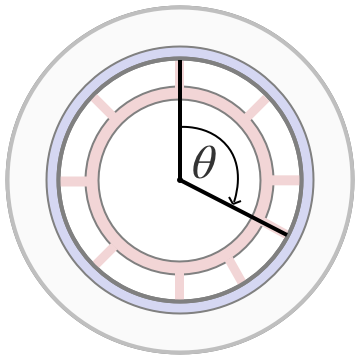## Optimizing Apple Park

And we’re back with another circle-themed geometry puzzle! This one’s about the new headquarters of Apple, Inc: Apple Park. (Remember, I don’t actually work in this building.)

Apple Park is a very big ring[citation needed]and there’s a corridor running along the inner part of the ring to get around it.There are also paved paths outside for when the weather’s nice, including one that goes all the way around in a circleand connects up with the building at several entrances.There are paths that cut through the middle too, but for this problem let’s assume there aren’t. The question is, if I’m trying to get partway around the ring, is it faster to stay insideor go outside and walk on the path?That’s the puzzle. Is it faster to walk around the corridor on the outer ring, or walk in to the path to walk around the inner ring?

Let’s define some terms to make this more straightforward. We’ll make $a$ and $b$ be the radii of the two rings (with $b$ being the bigger one)and $\theta$ be the angle around the ring that I’m trying to go.The formulas here are pretty simple: either I can walk directly around the corridor, a length of $b\theta$; or I can walk in $b-a$, around the path $a\theta$, and back out another $b-a$. Let’s find out the difference between the two.

Okay! So this tells us several things that make sense: the answer depends on the difference between the radii of the outer and inner rings, and on how far around I’m going to walk. That makes sense, because

• If the two rings are the same size $(a = b)$, there’s no difference between the two options.

• If there’s no inner ring $(a = 0)$ and I have to walk all the way to the middle, the choice is between $b\theta$ around the outside and just walking to the middle and back, $2b$.

• If I don’t go around the ring at all $(\theta = 0)$, it’s faster to not walk to the middle ring and then back to where I started.

At this point I took a step back and noticed that for the purposes of making this decision, it doesn’t matter what $a$ and $b$ are. All I want to know is which option is better, and that just means asking if $d$ is positive or negative.

That simplifies the problem. We know $% $ because otherwise $a$ wouldn’t be an “inner ring”. That in turn means that $b-a$ is always positive, and that means it won’t change the sign of $d$. So we can just ignore it.

And that gives us our answer. If $\theta$ is greater than 2 radians, then $d$ is positive, which means that walking around the corridor takes longer. And if $\theta$ is less than 2 radians, then walking in to the path takes longer.

Let’s check if the answer makes sense. I said above that if I don’t go around the ring at all $(\theta = 0)$, it’s faster to not walk to the middle ring and back. That matches this equation. And if I walk around and around 50 times, the time I save by walking a smaller circle is much more important than the very beginning and the very end where I walk in and out. So it seems right, and I trust the math. I have my answer; we could stop here. (Two radians is a bit less than a third of the way around the circle.)

But that “2” is a little funny. Where did that come from? It doesn’t even have τ in it.

Let’s take a look at that distance equation again.

Arranged this way, we have a different geometrical meaning for the two terms. Instead of thinking of $b-a$ as the difference between radii, let’s just say it’s a radius itself.That means we’re comparing the length of the arc, $\theta(b - a)$, with the length of the radius, twice. The “2” is just “walking these two straight lines”, and it’s okay to compare that to the length of the outside arc because that’s what a radian is. 1 radian is “the angle where the arc length is equal to the radius”, and so 2 radians is “the angle where the arc length is equal to twice the radius”. And that’s what I need to consider here, except it’s “the angle where the arc length is twice the difference in radii”.

I wrote that out and then paused. Why are those the same angle, two radians, in both cases?

…right, because changing the angle affects both circles evenly, or at least proportionally.

So, that’s our answer. And with that reminder of the definition of a radian, we can talk about “the number of radians that gives you an arc length that goes all the way around the circle”, and it turns out that’s 6.283…, or τ. The circumference of a circle $C$ is τ times the radius. We knew that.

Anyway, the right answer is to take the outside path unless it’s raining. It’s nice out there.